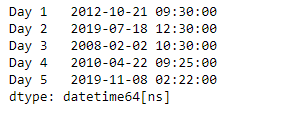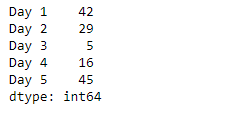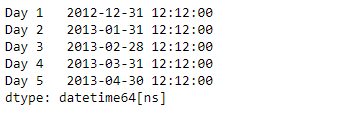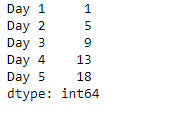# Python | Pandas Series.dt.week

• Last Updated : 20 Mar, 2019

`Series.dt` can be used to access the values of the series as datetimelike and return several properties. Pandas` Series.dt.week` attribute return a numpy array containing the week ordinal of the year in the underlying data of the given series object.

Syntax: Series.dt.week

Parameter : None

Returns : numpy array

Example #1: Use `Series.dt.week` attribute to return the week ordinal of the year in the underlying data of the given Series object.

 `# importing pandas as pd ` `import` `pandas as pd ` ` `  `# Creating the Series ` `sr ``=` `pd.Series([``'2012-10-21 09:30'``, ``'2019-7-18 12:30'``, ``'2008-02-2 10:30'``, ` `               ``'2010-4-22 09:25'``, ``'2019-11-8 02:22'``]) ` ` `  `# Creating the index ` `idx ``=` `[``'Day 1'``, ``'Day 2'``, ``'Day 3'``, ``'Day 4'``, ``'Day 5'``] ` ` `  `# set the index ` `sr.index ``=` `idx ` ` `  `# Convert the underlying data to datetime  ` `sr ``=` `pd.to_datetime(sr) ` ` `  `# Print the series ` `print``(sr) `

Output :Now we will use `Series.dt.week` attribute to return the week ordinal of the year in the underlying data of the given Series object.

 `# return the week ordinal ` `# of the year ` `result ``=` `sr.dt.week ` ` `  `# print the result ` `print``(result) `

Output :As we can see in the output, the `Series.dt.week` attribute has successfully accessed and returned the week ordinal of the year in the underlying data of the given series object.

Example #2 : Use `Series.dt.week` attribute to return the week ordinal of the year in the underlying data of the given Series object.

 `# importing pandas as pd ` `import` `pandas as pd ` ` `  `# Creating the Series ` `sr ``=` `pd.Series(pd.date_range(``'2012-12-12 12:12'``, periods ``=` `5``, freq ``=` `'M'``)) ` ` `  `# Creating the index ` `idx ``=` `[``'Day 1'``, ``'Day 2'``, ``'Day 3'``, ``'Day 4'``, ``'Day 5'``] ` ` `  `# set the index ` `sr.index ``=` `idx ` ` `  `# Print the series ` `print``(sr) `

Output :Now we will use `Series.dt.week` attribute to return the week ordinal of the year in the underlying data of the given Series object.

 `# return the week ordinal ` `# of the year ` `result ``=` `sr.dt.week ` ` `  `# print the result ` `print``(result) `

Output :As we can see in the output, the `Series.dt.week` attribute has successfully accessed and returned the week ordinal of the year in the underlying data of the given series object.

My Personal Notes arrow_drop_up
Recommended Articles
Page :